• 动态规划经典题目：包括了经典的最长不下降子序列，最长公共子序列，01背包，完全背包，部分背包等算法的详解。
• 题目：思路：先放上视频讲解动态规划经典题目：鸡蛋掉落https://www.zhihu.com/video/1225199247848513536纠正：视频里的状态转移方程漏写了一个+1，意思就是不管鸡蛋有没有摔碎，不管我们是往上走还是往下走，当前...
题目：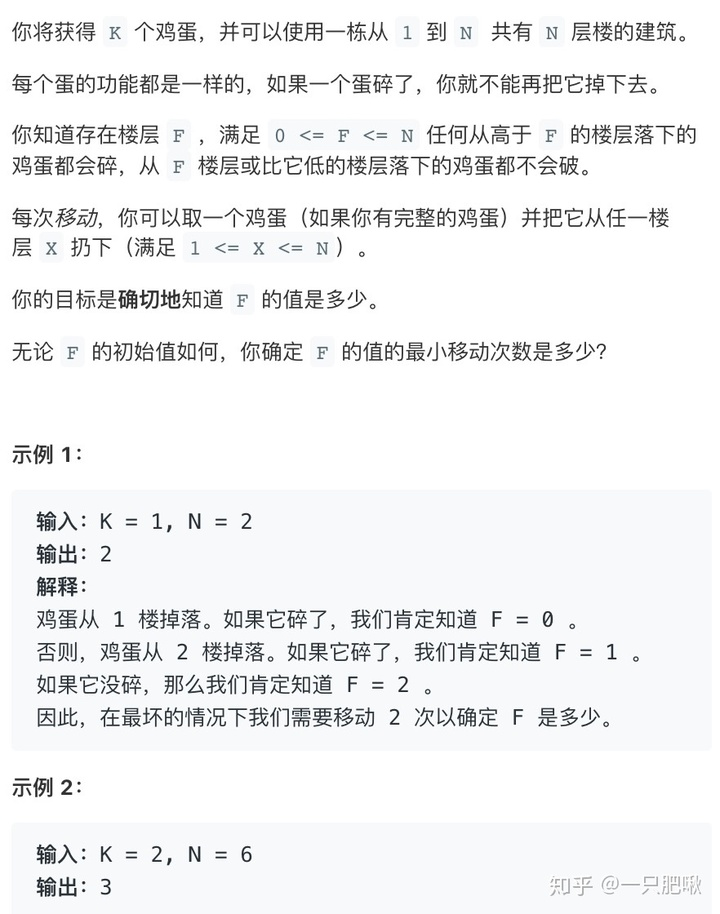思路：先放上视频讲解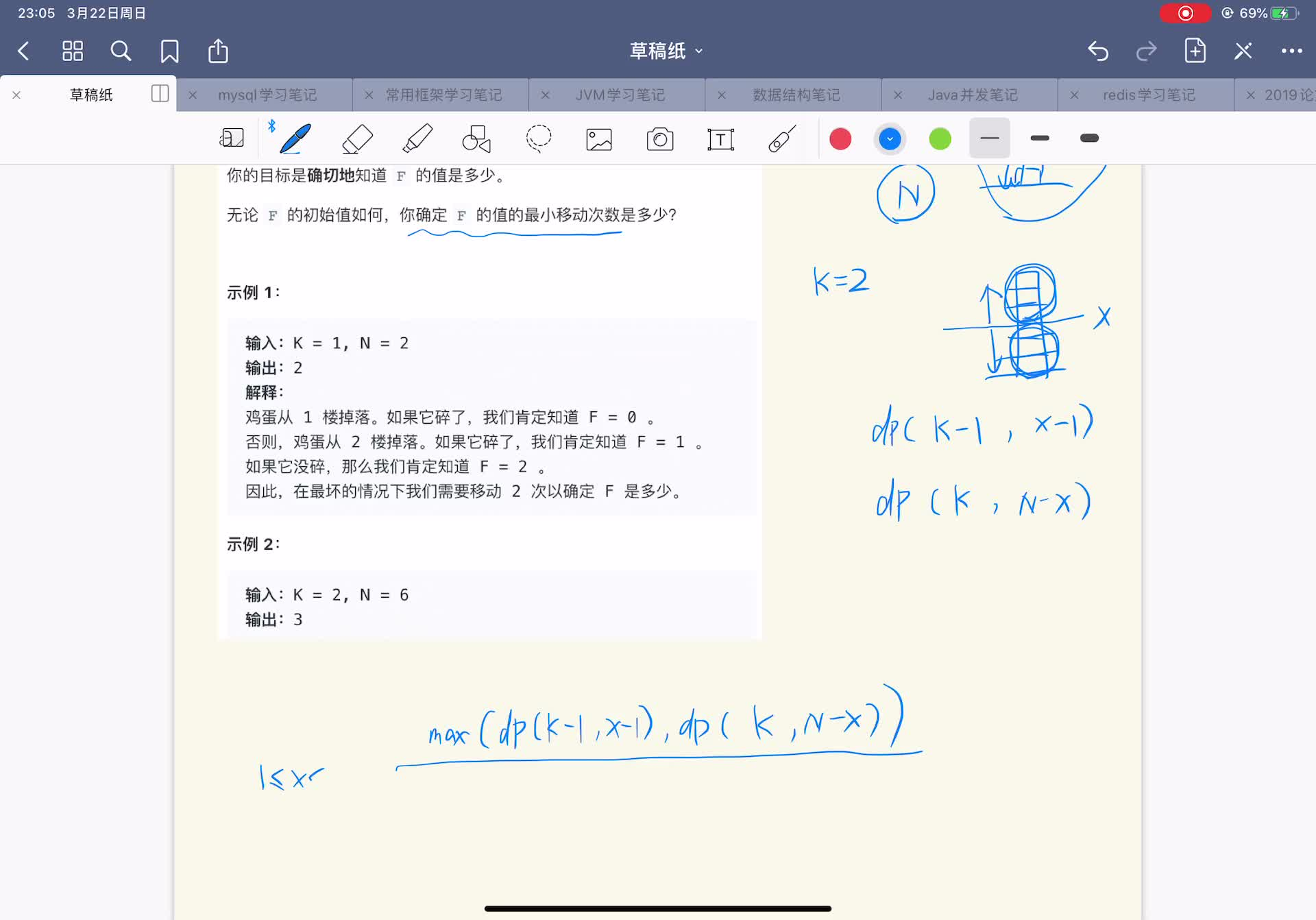动态规划经典题目：鸡蛋掉落https://www.zhihu.com/video/1225199247848513536纠正：视频里的状态转移方程漏写了一个+1，意思就是不管鸡蛋有没有摔碎，不管我们是往上走还是往下走，当前都已经扔过一次了，所以次数+1。代码（优化前）：class Solution {
public int superEggDrop(int K, int N) {
//dp存的是拥有i个鸡蛋j层楼情况下找到F所需的最小移动次数
int[][] dp = new int[K+1][N+1];
//只有一个鸡蛋时，最坏需要移动（当前拥有的楼层数）次
for(int i=0;i<=N;i++){
dp[i] = i;
}
//从两个鸡蛋的情况开始算
for(int i=2;i<=K;i++){
//在拥有i个鸡蛋j个楼层的情况下，计算从h层扔鸡蛋去找F层所需的移动次数
for(int j=1;j<=N;j++){
int ans = Integer.MAX_VALUE;
for(int h=1;h<=j;h++){
int A = dp[i-1][h-1];
int B = dp[i][j-h];
//ans为h从1取到j层楼的所有情况里的最小移动次数
ans = Math.min(ans,Math.max(A,B)+1);
}
dp[i][j] = ans;
}
}
return dp[K][N];
}
}这是结合我视频里的思路写出来的代码，比较便于理解。但是由于h一次次地从1取到当前拥有的楼层数，然后才能比较出最小值，这中间做了很多不必要的工作，所以还需要进行优化。回头看一下这个状态转移方程：max(dp(K-1,X-1),dp(K,N-X))可以发现：当X增大时，dp(K-1,X-1)是随之增大的。这也很好理解，楼层越多，想找到F就需要更多的次数。而当X增大时，dp(K,N-X)是随之减小的。和上条是一样的道理，X越大，N-X就越小，即楼层越少，那么找到F所需的次数就更少。而max(dp(K-1,X-1),dp(K,N-X))，取的是两者中的较大数。画个图理解一下（当然真实数据并不是图片所展示的这样，这个图只是为了便于理解）：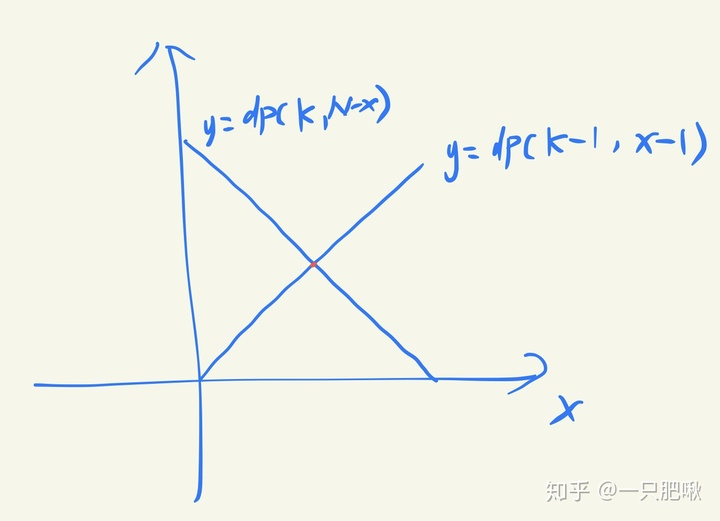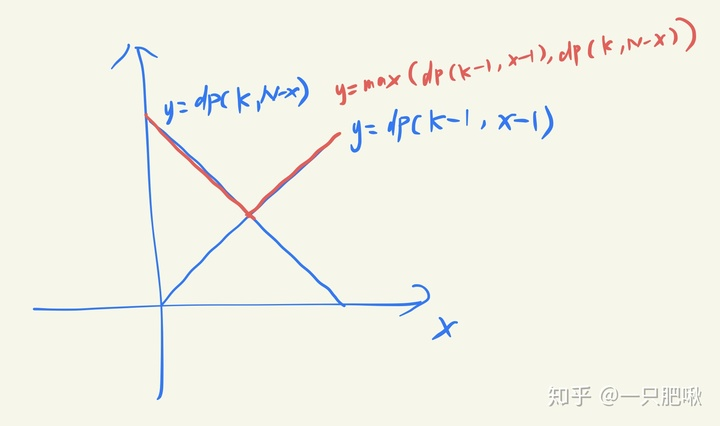红色折线即为max(dp(K-1,X-1),dp(K,N-X))的图像所以max(dp(K-1,X-1),dp(K,N-X))是先递减后递增的，它的最小值即为我们要找的最小移动次数。也就是说，我们不必每次都让h从1开始遍历完所有的楼层，只要找到一个X，使得                    max(dp(K-1,X-1),dp(K,N-X))<max(dp(K-1,(X+1)-1),dp(K,N-(X+1)))成立，那么这个X就是让max取到最小值时的层数。代码（优化后）：class Solution {
public int superEggDrop(int K, int N) {
//dp存的是拥有i个鸡蛋j层楼情况下找到F所需的最小移动次数
int[][] dp = new int[K+1][N+1];
//只有一个鸡蛋时，最坏需要移动（当前拥有的楼层数）次
for(int i=0;i<=N;i++){
dp[i] = i;
}
//从两个鸡蛋的情况开始算
for(int i=2;i<=K;i++){
int x=1;
for(int j=1;j<=N;j++){
//当取x层比取X+1层的结果要大时，x++（再往上找）
while(x<j && Math.max(dp[i-1][x-1],dp[i][j-x])>Math.max(dp[i-1][x],dp[i][j-x-1]))
x++;
dp[i][j] = Math.max(dp[i-1][x-1],dp[i][j-x])+1;
}
}
return dp[K][N];
}
}总结：动态规划就是一个自底向上计算的过程，保存每个前面的值，便于后面去计算。像这道题，我们先算好K=1的情况，便于给K=2的情况用。接下来，一步步根据前面的值算出dp，dp……就这样一直自底向上计算出dp[K][N]。补充：经评论区大神指点，发现还可以做另一种优化。像上面分析的那样，dp(K-1,X-1)是递增函数，dp(K,N-X)是递减函数，max在两者相交时取到最小值。如下图所示。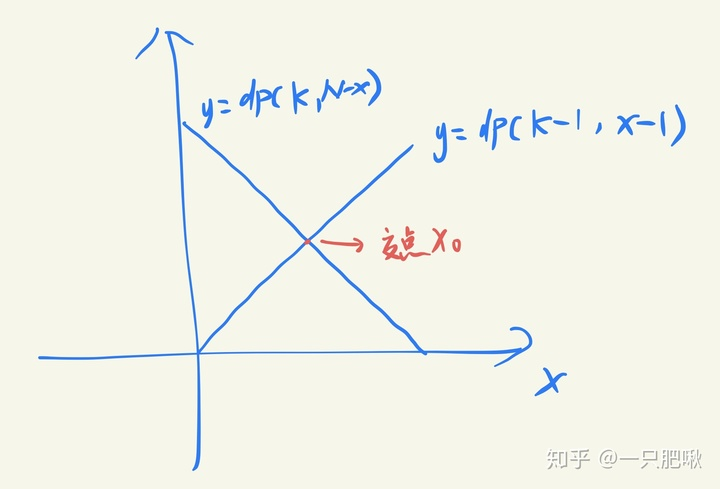那么我们令low=1，high=N（当前总楼层数），index=(low+high)/2，根据以上图像，我们会发现：如果dp(K-1,index-1)>dp(K,N-index)，说明当前index位于交点右侧，可以缩小查找区间至[low,index]，否则说明index位于交点的左侧，缩小查找区间为[index,high]。根据这个规律，就可以通过二分法去查找到这个交点了！代码（优化二）：class Solution {
public int superEggDrop(int K, int N) {
//dp存的是拥有i个鸡蛋j层楼情况下找到F所需的最小移动次数
int[][] dp = new int[K+1][N+1];
//只有一个鸡蛋时，最坏需要移动（当前拥有的楼层数）次
for(int i=0;i<=N;i++){
dp[i] = i;
}
//从两个鸡蛋的情况开始算
for(int i=2;i<=K;i++){
for(int j=1;j<=N;j++){
//通过二分法去查找能取到最小移动次数的层数
int low=1,high=j;
while(low+1<high){
int index = (low+high)/2;
int A = dp[i-1][index-1];
int B = dp[i][j-index];
if(A>B){
high = index;
}else if(A<B){
low = index;
}else{
low = high = index;
}
}
//dp[i][j]=min(选择low层时的移动次数,选择high层时的移动次数)
dp[i][j] = Math.min(Math.max(dp[i-1][low-1],dp[i][j-low]),Math.max(dp[i-1][high-1],dp[i][j-high]))+1;
}
}
return dp[K][N];
}
}注意：这个交点可能并不是整数（无法取到这个楼层），所以最后要用low和high分别代入计算，再取最小值。最后的一点感想：终于写完了。。。曾经让我望而却步的算法，现在也能轻松掌握了，还是很有成就感的~其实学这些真的没有那么难，只要多一点耐心和毅力，相信自己就好。最后借用添哥一句话吧：我无坚不摧，也无所不能。与君共勉~
展开全文• ## 动态规划经典题目总结

万次阅读 多人点赞 2018-12-27 11:31:30
在算法中，动态规划题目算是比较经典的一类题目。在找工作中，不管是笔试，还是面试，我们经常会遇到用动态规划来解决问题的情况，有时候面试官还需要我们现场手写出动态规划解法的代码。因此，在求职中能灵活的运用...


在算法中，动态规划题目算是比较经典的一类题目。在找工作中，不管是笔试，还是面试，我们经常会遇到用动态规划来解决问题的情况，有时候面试官还需要我们现场手写出动态规划解法的代码。因此，在求职中能灵活的运用动态规划就相当重要了。下面我总结出了一些经典的动态规划题目，其中有些还是面试中遇到的。

1. 什么是动态规划

【1】牛客网在线编程专题《剑指offer-面试题9》斐波那契数列

【2】动态规划学习-【国王和金矿】

2. 第一个动态规划问题 Climbing Stairs

【1】牛客网在线编程专题《剑指offer-面试题9：题目二》跳台阶

【2】【LeetCode】70. Climbing Stairs

【3】【LeetCode】120. Triangle

【4】【LeetCode】64. Minimum Path Sum

3. 发现重叠子问题 Integer Break

【1】【LeetCode】343. Integer Break

【2】【LeetCode】279. Perfect Squares

【3】【LeetCode】91. Decode Ways

【4】【LeetCode】62. Unique Paths

【5】【LeetCode】63. Unique Paths II

4. 状态的定义和状态转移 House Robber

【1】【LeetCode】198. House Robber

【2】【LeetCode】213. House Robber ||

【3】【LeetCode】337. House Robber |||

【4】【LeetCode-面试-算法】股票的最大盈利值

【5】【LeetCode】309. Best Time to Buy and Sell Stock with Cooldown

5. 阶段练习

【1】【动态规划】Subarray Sum Equals K-子数组和为K

【2】【动态规划】求数组不相邻元素之和最大

【3】牛客网在线编程专题《剑指offer-面试题31》连续子数组的最大和

【4】【LeetCode】53. Maximum Subarray

【5】牛客网在线编程专题《剑指offer-面试题9：相关题目》矩形覆盖

6. 0-1背包问题

【1】动态规划学习-【0-1背包问题】

7. 0-1背包问题的优化和变种

【1】动态规划学习-【0-1背包问题的优化和变种】

8. 面试中的0-1背包问题 Partition Equal Subset Sum

【1】【LeetCode】416. Partition Equal Subset Sum

【2】【LeetCode】322. Coin Change

【3】【LeetCode】377. Combination Sum IV

【4】【LeetCode】474. Ones and Zeroes

【5】【LeetCode】139. Word Break

【6】【LeetCode】494. Target Sum

9. LIS问题 Longest Increasing Subsequence

【1】【LeetCode】300. Longest Increasing Subsequence

【2】【LeetCode】376. Wiggle Subsequence

10. LCS、最短路径、求动态规划的具体解以及更多

【1】【LeetCode】最长公共子序列 | 718. Maximum Length of Repeated Subarray | 最短路径

11. 面试中常考的经典动态规划题目

【1】【LeetCode】72. Edit Distance

展开全文算法 LeetCode 剑指offer
• 动态规划经典题目及解答（含代码pdf） 1． 最长公共子序列 2． 计算矩阵连乘积 3． 凸多边形的最优三角剖分 4． 防卫导弹 5． 石子合并 6． 最小代价子母树 7． 商店购物 8． 旅游预算 9． 皇宫看守 10． 游戏室问题...
• 针对最常见的最优化问题，动态规划如何设计求解呢？下面我们研究一个最优化问题：矿工挖矿问题。矿工挖矿问题是为了解决在给定矿产和矿工数量的前提下，能够获得最多钻石的挖矿策略。1. 问题描述假设某地区有 5 座...
针对最常见的最优化问题，动态规划如何设计求解呢？下面我们研究一个最优化问题：矿工挖矿问题。矿工挖矿问题是为了解决在给定矿产和矿工数量的前提下，能够获得最多钻石的挖矿策略。1. 问题描述假设某地区有 5 座钻石矿，每座钻石矿的钻石储量不同，根据挖矿难度，需要参与挖掘的工人数量也不同。假设能够参与挖矿工人的总数是 10 人，且每座钻石矿要么全挖，要么不挖，不能只派出一部分人挖取一部分矿产。要求用程序求解出，要想得到尽可能多的钻石，应该选择挖取哪几座矿产？各矿产的钻石储量与所需挖掘工人数量如表 1 所示。表 1：各矿产的钻石储量与所需工人数量矿产编号钻石储量所需工人数量14005250053200343004535032. 问题分析1)分析原问题最优解的结构特征首先寻找最优子结构。我们的解题目标是确定 10 个工人挖 5 座矿产时能够获得的最多的钻石数量，该结果可以从 10 个工人挖 4 个矿产的子问题中递归求解。证明不再赘述。在解决了 10 个工人挖 4 个矿产后，存在两种选择：一种选择是放弃其中一座矿，比如第五座矿产，将 10 个工人全部投放到前4座矿产的挖掘中，如图 1 所示；图 1：放弃第 5 座矿产的情况另一种选择是对第 5 座矿产进行挖掘，因此需要从 10 人中分配 3 个人加入到第 5 座矿产的挖掘工作中，如图 2 所示。图 2：挖掘第 5 座矿产的情况因此，最终的最优解应该是这两种选择中获得钻石数量较多的那个，即为图 1 所描述的场景与图 2 所描述场景中的最大值。为了方便描述，我们假设矿产的数量为 n，工人的数量为 m，当前获得的钻石数量为 G[n]，当前所用矿工的数量为 L[n]，则根据上述分析，要获得 10 个矿工挖掘第 5 座矿产的最优解 F(5,10)，需要在 F(4,10)和F(4,10-L)+G 中获取较大的值，即F(5,10)=max{F(4,10),F(4,10-L)+G}因此，针对该问题而言，以上便是 F(5,10) 情况下的最优子结构。2) 建立递归关系，写出状态转移函数我们首先来考虑该问题的边界和初始条件。对于一个矿产的情况，若当前的矿工数量不能满足该矿产的挖掘需要，则获得的钻石数量为 0，若能满足矿工数量要求，则获得的钻石数量为 G。因此，该问题的初始边界条件可表述为：当n=1,m≥L时，F(n,m)=G；当n=1,m综上，可以得到该问题的状态转移函数为：F(n,m)=0(n≤1,mF(n,m)=G(n==1,m≥L)F(n,m)=F(n-1,m)(n>1,mF(n,m)=max(F(n-1,m),F(n-1,m-L[n-1])+G[n-1])(n>1,m≥L[n-1])至此，我们定义了用动态规划解决该问题的几个要素。下面，我们要做的是利用边界和初始条件、最优子结构和状态转移函数对该问题进行求解。3) 计算最优解的值初始化阶段，我们利用表格分析求解思路。如表 2 所示，表格的第一列代表挖掘矿产数，即 n 的取值情况；表格的第一行代表占用工人数，即 m 的取值情况；中间各空白区域是我们需要通过计算填入的对应的钻石数量，即 F(n,m) 的取值。表 2：初始钻石数量矿产编号 nm=1 人m=2 人m=3 人m=4 人m=5 人m=6 人m=7 人m=8 人m=9 人m=10 人矿产 1矿产 2矿产 3矿产 4矿产 5在挖掘第一个矿产时，由于其所需的工人数量为 5，所以当 m 的取值小于 5 时，根据公式 F(n,m)=0(n≤1,m表 3：挖掘第1个矿产时钻石数量矿产编号 nm=1 人m=2 人m=3 人m=4 人m=5 人m=6 人m=7 人m=8 人m=9 人m=10 人矿产 10000400400400400400400矿产 2矿产 3矿产 4矿产 5在挖掘第 2 个矿产时，由于其需要 5 个人进行挖掘，因此当 m 取值小于 5 时，根据公式 F(n,m)=F(n-1,m)(n>1,m1,m≥L[n-1])，在 5～9 人的区间里，获得的钻石数量为 500，即所有人都去参加第 2 个矿产的挖掘时获得的钻石量。这是因为当 m∈{5,9}时， F(1,m)表 4：挖掘第 2 个矿产时钻石数量矿产编号 nm=1 人m=2 人m=3 人m=4 人m=5 人m=6 人m=7 人m=8 人m=9 人m=10 人矿产 10000400400400400400400矿产 20000500500500500500500矿产 3矿产 4矿产 5同理，在挖掘第 3 个矿产时，钻石产出量为 200，需要的工人数量为 3，根据上述计算方式，可得钻石产出量如表 5 所示。表 5：挖掘第 3 个矿产时钻石数量矿产编号 nm=1 人m=2 人m=3 人m=4 人m=5 人m=6 人m=7 人m=8 人m=9 人m=10 人矿产 10000400400400400400400矿产 20000500500500500500500矿产 300200200500500500700700900矿产 4矿产 5第 4 个矿产的钻石产出量为 300，需要的工人数量为 4，根据上述计算方式，可得钻石产出量如表 6 所示。表 6：挖掘第 4 个矿产时钻石数量矿产编号 nm=1 人m=2 人m=3 人m=4 人m=5 人m=6 人m=7 人m=8 人m=9 人m=10 人矿产 10000400400400400400400矿产 20000500500500500500500矿产 300200200500500500700700900矿产 400200300500500500700800900矿产 5针对第 5 个矿产的钻石产出量计算与上述过程一致，具体产出量如表 7 所示。表 7：挖掘第 5 个矿产时钻石数量矿产编号 nm=1 人m=2 人m=3 人m=4 人m=5 人m=6 人m=7 人m=8 人m=9 人m=10 人矿产 10000400400400400400400矿产 20000500500500500500500矿产 300200200500500500700700900矿产 400200300500500500700800900矿产 500350350500550650850850900通过以上的计算过程，我们不难发现，除第一个矿产的相关数据，表格中的其他数据都可以由前一行的一个或两个格子推导而来。例如，3 个矿产 8 个人挖掘的钻石量 F(3,8) 就来自 2 个矿产 8 个人挖掘的钻石量 F(2,8)和 2 个矿产 5 个人挖掘的钻石量 F(2,5)，即 F(3,8)=max{F(2,8),F(2,5)+200}=max(500,500+200)=700。再比如，4 个矿产 10 个人挖掘的钻石量 F(4,10)，来自 3 个矿产 10 个人挖掘的钻石量 F(3,10)和 3 个矿产 7 个人挖掘的钻石量 F(3,7)，即 F(4,10)=max{F(3,10)，F(3,7)+300}=max(900， 500+300)=900，如表 8 所示。表 8：矿产钻石产量计算依赖于之前计算量矿产编号 nm=1 人m=2 人m=3 人m=4 人m=5 人m=6 人m=7 人m=8 人m=9 人m=10 人矿产 10000400400400400400400矿产 20000500500500500500500矿产 3矿产 4矿产 5根据以上思路，在用程序实现该算法的过程中，采用自底向上的方式进行计算，像填表过程一样从左至右、从上到下逐渐获得计算结果。这样，可以不需要存储整个表格的内容，仅需要存储前一行的结果，就可以推出下一行的内容，避免了重复计算。4) 构造最优解填表结束后，我们通过回溯的方式可以找出该矿工挖矿问题的最优解组合为所有矿工(10人)挖掘矿产 1 和矿产 2，最多可以挖得价值 900 的钻石。具体回溯过程我们之后展示。3. 参考实现现在我们来把这个过程转化为程序。我们定义函数 goldMine(n,m,g,L) 来计算挖掘第 n 个矿产，有 m 个工人参与时能获得的钻石量，其中 g 和 L 分别为数组，分别存放对应各个矿产的钻石量和所需工人数。在正式迭代之前，首先界定边界的情况。当工人数量小于 L时，说明目前的工人数量不能开始任何矿产的挖掘，获得的钻石数量为 0；当工人数量大于或等于 L时，获得的钻石数量即为 g。之后进入循环的迭代过程，在迭代中，根据之前的分析，我们仅需要关注前一行 t_results 的取值，即可通过状态转移函数 F(n,m)=F(n-1,m)(n>1,m1,m≥L[n-1]) 获得 F(n,m) 的值，因此，整个迭代过程仅需要引入 t_results 数组保存前一行的值即可。由此可见，该解决方案的时间复杂度为 O(ni m)，而空间复杂度只有 O(m)。具体实现时，我们首先定义边界值，确定数组 t_results 各个元素的取值，同时初始化数组 results。之后，通过函数 goldMine(n,m,g,L) 迭代计算各个矿产数量与工人数量组合所能产生的钻石量，最终获得问题的解。矿工挖矿问题代码如下：def goldMine(n, m, g, L):results = [0 for _ in range(m+1)]　　　　 #保存返回结果的数组t_results = [0 for _ in range(m+1)]　　　　#保存上一行结果的数组for i in range(1,m+1):　　　 #填充边界格子的值，从左向右填充表格第一行的内容if　i < L:t_results[i] = 0#若当前人数少于挖掘第一个金矿所需人数，黄金量为0else:t_results[i] = g　#若当前人数不少于第一个金矿所需人数，黄金量为gfor i in range(1,n):　　　　　　 #外层循环为金矿数量results = [0 for _ in range(m+1)]for j in range(1,m+1):　　 #内层循环为矿工数量if j < L[i]:results[j] = t_results[j]else:results[j] = max(t_results[j], t_results[j-L[i]] + g[i])t_results = resultsreturn results[-1]print(goldMine(5, 10, [400,500,200,300,350], [5,5,3,4,3]))输出：900
展开全文• 动态规划经典题目整理背包问题最长公共子串问题连续数组最大和问题 背包问题 复杂度 o(nW) n为物品种类，W是背包的重量 单副本背包问题： K(w,j)=max{K(w−wj,j−1)+vj,K(w,j−1)}K(w,j)=max\{K(w-w_j,j-1)+v_j,K(w,...
动态规划经典题目整理背包问题最长公共子串问题连续数组最大和问题持续增加中。。。。
背包问题
复杂度 $O(nW)$
$n$为物品种类，$W$是背包的重量
目的：使得背包中的物品价值最大化

单副本背包问题：（每种物品只有一件）
$K(w,j)=max\{K(w-w_j,j-1)+v_j,K(w,j-1)\}$
$K(w,j)$代表背包重量为$W$，有$j$件物品时候的最大价值
$v_j$为第$j$种物品的价值

多副本背包问题：（每种物品有无数件）
$K(w)=max\{K(w-w_i)+v_i:w_i<=w\}$

最长公共子串问题
博客入口
问题描述： 设有$s[m]$，和$t[n]$两个字符串，要找他们最长的公共子串
解：
设置一个变量 存储最长公共子串的长度
long=0;
c(i,j)=s[i]==s[j]?c(i-1,j-1)+1:0
每次如果 c(i,j)>long 那么 long=c(i,j)
设两个子序列的长度分别为$m$,$n$
那么时间复杂度就为$O(mn)$
连续数组最大和问题
例子：
给定一个数组如：
{-2, 11, -4, 13, -5, -2 }
该数组的最大和为 i=2, j=4的时候 11-4+13=20
算法:
int sum=0, b=0;

for(int i=1;i<=n;++i){
if(b>0){
b+=a[i];
}
else{
b=a[i]
}
if(b>sum)
sum=b;
}
return sum;

复杂度：$O(n)$
持续增加中。。。。


展开全文• 动态规划经典题目加总结 动态规划理解：将子问题用递推的方法，并且将中间结果保留以避免重复计算的方法就叫做动态规划，动态规划通常用来求最优解能够用动态规划求最优解的问题必须满足：最优解的每个局部都是最优d...
• 动态规划经典题目及解答，经典的！！常用的题目分析，可以让你更好掌握~~~~~~~
• 此题的解法与动态规划经典题目——最大连续子序列之和题目思想一样，只不过本题是二维空间上的拓展。N*N矩阵用二维数组a[N][N]表示。我们解决新问题的方法是借鉴我们以前解决过的类似问题的方法和思路，当然本题也...最大子矩阵和
• 分割等和子集-----------上篇文章 经典动态规划：0-1 背包问题 详解了通用的 0-1 背包问题，今天来看看背包问题的思想能够如何运用到其他算法题目。而且，不是经常有读者问，怎么将二维动态规划压缩成一维动态规划吗...
• 最小路径和(Medium)挺久没写动态规划的文章了，今天聊一道经典动态规划题目，最小路径和。它是力扣第 64 题，我来简单描述一下题目：现在给你输入一个二维数组grid，其中的元素都是非负整数，现在你站在左上角，...
• 爬楼梯和斐波那契很像，但相比昨天的题目可就有难度了，来看看难到哪里了！ 通知：我已经将刷题攻略全部整理到了Github ：https://github.com/youngyangyang04/leetcode-master，方便大家在电脑上阅读，这个仓库每天...
• 许多的动态规划经典题目,有大牛的分析和解题思路,对于中级OI选手十分的有用.
• 通知：我已经将刷题指南全部整理到...周一「力扣」动态规划经典面试题：不同路径 中求从出发点到终点有几种路径，只能向下或者向右移动一步。我们提供了三种方法，但重点讲解的还是动规，也是需要重点掌握的。dp[i]...
• 如果你对最长公共子序列(LCS)题目不太了解，可以回顾之前的文章： LeetCode 例题精讲 | 15 最长公共子序列：二维动态规划的解法 我用 LCS 作为二维动态规划的例题，就是因为它的方法非常经典，很多其他题目中都有它...
• 给定一个无序的整数数组，找到其中最长上升子序列的长度。示例:输入: [10,9,2,5,3,7,101,18]输出: 4解释: 最长的上升子序列是[2,3,7,101]，它的长度是 4。说明:可能会有多种最长上升子序列的组合，你只需要输出对应...
• 关于动态规划的一些经典题目，有助于提高编程能力，对于学习有很大的准备，，！！！期待大家学习分享！
• 动态规划入门请看： DP动态规划专题（一）动态规划基本模型 前言 【说明】 关于动态规划的见解：动规和递归有很多相似的地方，最显著的特征可以说是阶段性，二者都有很明显的阶段划分，所以，声明好每一个阶段......